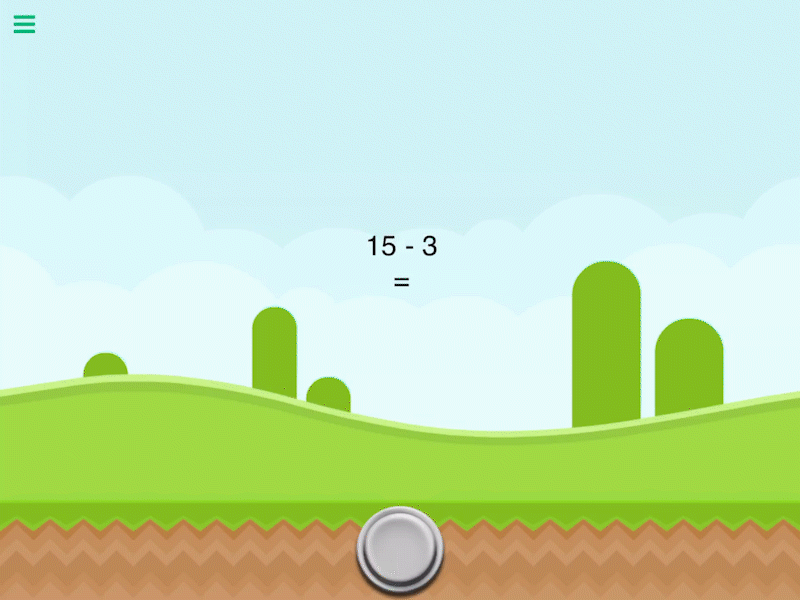# Subtract Values

Description

Subtract a number from another number. This will output the difference of the 2 numbers entered

Outputs

 Subtract Values Outputs the difference of two inputs.

Examples

4 subtract 2 outputs 2.

Related

Divide Values

Modulus

Multiply Values